Home » How to Improve Class Imbalance using Class Weights in Machine Learning

# How to Improve Class Imbalance using Class Weights in Machine Learning

This article was published as a part of the Data Science Blogathon.

## Overview

• Understand how class weight optimization works and how we can implement the same in logistic regression or any other algorithm using sklearn
• Learn how class weights can help overcome the class imbalance data problems without using any sampling method

## Introduction

A classification problem in machine learning is where we have given some input (independent variables), and we have to predict a discrete target. It is highly possible that the distribution of discrete values will be very different. Due to this difference in each class, the algorithms tend to get biased towards the majority values present and don’t perform well on the minority values.

This difference in class frequencies affects the overall predictability of the model.

Getting good accuracy on these problems is not very difficult, but it is not always about getting a good score. We need to check whether the performance of these models makes any business sense or have any value. That is why it is essential to understand your problem statement and data so that you could use the right metric and optimize it using suitable methods.

• What is class imbalance?
• Why is it essential to deal with class imbalance?
• What are the class weights?
• Class weights in logistic regression
• Python implementation
• Simple logistic regression
• Weighted logistic regression(‘balanced’)
• Weighted logistic regression(manual weights)
• Tips to improve scores further

## What is Class Imbalance?

Class imbalance is a problem that occurs in machine learning classification problems. It merely tells that the target class’s frequency is highly imbalanced, i.e., the occurrence of one of the classes is very high compared to the other classes present. In other words, there is a bias or skewness towards the majority class present in the target. Suppose we consider a binary classification where the majority target class has 10000 rows, and the minority target class has only 100 rows. In that case, the ratio is 100:1, i.e., for every 100 majority class, there is only one minority class present. This problem is what we refer to as class imbalance. Some of the general areas where we can find such data are fraud detection, churn prediction, medical diagnosis, e-mail classification, etc.

We will be working on a dataset from the medical domain to understand class imbalance properly. Here, we have to predict whether a person will have a heart stroke or not based on the given attributes(independent variables). To skip the cleaning and the preprocessing of the data, we are using the cleaned version of the data.

In the below image, you can see the distribution of our target variable.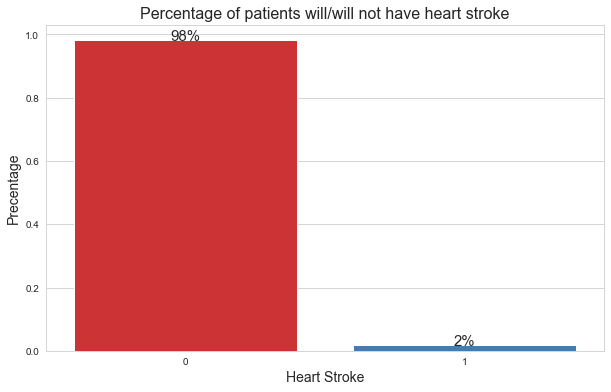Here,

0: signifies that the patient didn’t have a heart stroke.

1: signifies that the patient had a heart stroke.

From the distribution, we can see that there are only 2% of patients who had a heart stroke. So, this is a classic class imbalance problem.

## Why is it essential to deal with class imbalance?

So far, we got the intuition about class imbalance. But why is it necessary to overcome this, and what problems does it create while modeling with such data?

Most machine learning algorithms assume that the data is evenly distributed within classes. In the case of class imbalance problems, the extensive issue is that the algorithm will be more biased towards predicting the majority class (no heart stroke in our case). The algorithm will not have enough data to learn the patterns present in the minority class (heart stroke).

Let’s take a real-life example to understand this better.

Consider you have shifted from your hometown to a new city and you been living here for the past month. When it comes to your hometown, you will be very familiar with all the locations like your home, routes, essential shops, tourist spots, etc. because you had spent your whole childhood there. But when it comes to the new city, you would not have many ideas about where each location exactly is, and the chances of taking the wrong routes and getting lost will be very high. Here, your hometown is your majority class, and the new city is the minority class.

Similarly, this happens in class imbalance. The model has adequate information about the majority class but insufficient information about your minority class. That is why there will be high misclassification errors for the minority class.

Note: To check the performance of the model, we will be using the f1 score as the metric, not accuracy.

The reason is if we create a dumb model that predicts every new training data as 0 (no heart stroke) even then we will get very high accuracy because the model is biased towards the majority class. Here, the model is heavily accurate but not at all serving any value to our problem statement. That is why we will be using f1 score as the evaluation metric. F1 score is nothing but the harmonic mean of precision and recall. However, the evaluation metric is chosen based on the business problem and what type of error we want to reduce. But, the f1 score is the go-to metric when it comes to class imbalance problems.

Here’s the formula for f1-score:

f1 score = 2*(precision*recall)/(precision+recall)

Let’s confirm this by training a model based on the mode of the target variable on our heart stroke data and check what scores we get:

The accuracy for the mode model is: 0.9819508448540707

The f1 score for the mode model is: 0.0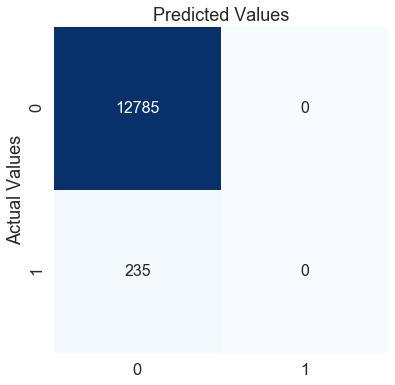Here, the accuracy of the mode model on the testing data is 0.98 which is an excellent score. But on the other hand, the f1 score is zero which indicates that the model is performing poorly on the minority class. We can confirm this by looking at the confusion matrix.

The mode model is predicting every patient as 0 (no heart stroke). According to this model, no matter what the symptoms a patient has, he/she will never have a heart stroke. Does using this model makes any sense?

Now that we got the gist of what is class imbalance and how it plagues our model performance, we will shift our focus to what class weights are and how class weights can help in improving the model performance.

## What are the class weights?

Most machine learning algorithms are not very useful with biased class data. But, we can modify the current training algorithm to take into account the skewed distribution of the classes. This can be achieved by giving different weights to both the majority and minority classes. The difference in weights will influence the classification of the classes during the training phase. The whole purpose is to penalize the misclassification made by the minority class by setting a higher class weight and at the same time reducing weight for the majority class.

To make this a bit clear, we will be reviving the city example we considered earlier.

Please think of it this way that the last month you have spent in the new city, instead of going out when it is needed, you spent the whole month exploring the city. You spent more time understanding the city routes and places the entire month. Giving more time to research will help you to understand the new city better, and the chances of getting lost will reduce. And this is precisely how class weights work. During the training, we give more weightage to the minority class in the cost function of the algorithm so that it could provide a higher penalty to the minority class and the algorithm could focus on reducing the errors for the minority class.

Note: There is a threshold to which you should increase and decrease the class weights for the minority and majority class respectively. If you give very high-class weights to the minority class, chances are the algorithm will get biased towards the minority class, and it will increase the errors in the majority class.

Most of the sklearn classifier modeling libraries and even some boosting based libraries like LightGBM and catboost have an in-built parameter “class_weight” which helps us optimize the scoring for the minority class just the way we have learned so far.

By default, the value of class_weight=None, i.e. both the classes have been given equal weights. Other than that, we can either give it as ‘balanced’ or we can pass a dictionary that contains manual weights for both the classes.

When the class_weights = ‘balanced’, the model automatically assigns the class weights inversely proportional to their respective frequencies.

To be more precise, the formula to calculate this is:

wj=n_samples / (n_classes * n_samplesj)

Here,

• wj is the weight for each class(j signifies the class)
• n_samplesis the total number of samples or rows in the dataset
• n_classesis the total number of unique classes in the target
• n_samplesjis the total number of rows of the respective class

For our heart stroke example:

n_samples=  43400,  n_classes= 2(0&1), n_sample0= 42617, n_samples1= 783

Weights for class 0:

w0=  43400/(2*42617) = 0.509

Weights for class 1:

w1= 43400/(2*783) = 27.713

I hope this makes things more clear that how class_weight = ‘balanced’ helps us to in giving higher weights to the minority class and lower weights to the majority class.

Although passing value as ‘balanced’ gives good results in most cases but sometimes for extreme class imbalance, we can try giving weights manually. Later we will see how we can find the optimal value for the class weights in Python.

## Class weights in Logistic Regression

We can modify every machine learning algorithm by adding different class weights to the cost function of the algorithm, but here we will specifically focus on logistic regression.

For the logistic regression, we use log loss as the cost function. We don’t use the mean squared error as the cost function for the logistic regression because instead of fitting a straight line, we use the sigmoid curve as the prediction function. Squaring the sigmoid function will result in a non-convex curve due to which the cost function will have a lot of local minima and converging to the global minima using gradient descent is extremely difficult. But log loss forms a convex function, and we only have one minimum to converge.

The formula for log loss: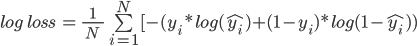Here,

• N is the number of values
• yi is the actual value of the target class
• yi is the predicted probability of the target class

Let’s form a pseudo table that has actual predictions, predicted probabilities, and calculated cost using the log loss formula: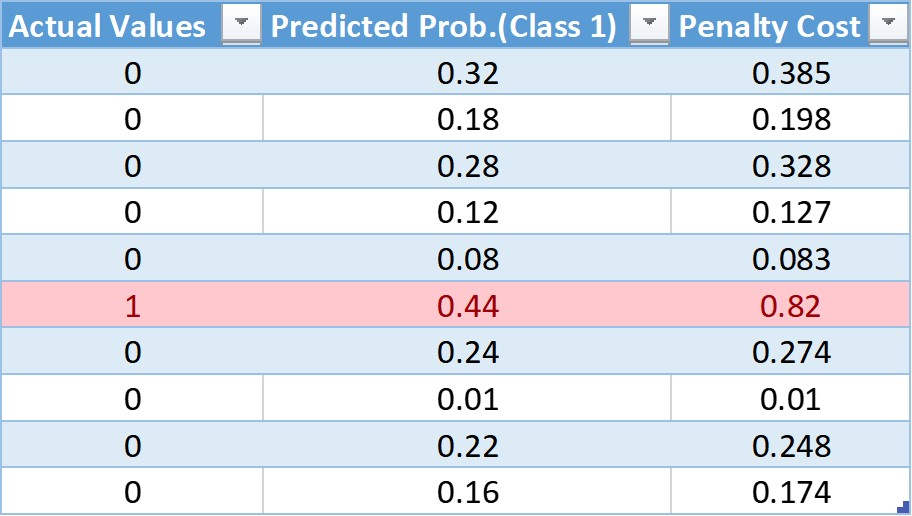In this table, we have ten observations with nine observations from class 0 and 1 from class 1. In the next column, we have the predicted probabilities for each observation. And finally, using the log loss formula, we have the cost penalty.

After adding the weights to the cost function, the modified log loss function is: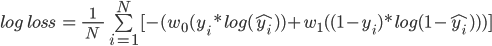Here,

• w0 is the class weight for class 0
• w1 is the class weight for class 1

Now, we will add the weights and see what difference will it make to the cost penalty.

For the values of the weights, we will be using the class_weights=’balanced’ formula.

w0= 10/(2*1) = 5

w1= 10/(2*9) = 0.55

Calculating the cost for the first value in the table:

Cost = -(5(0*log(0.32) + 0.55(1-0)*log(1-0.32))

= -(0 + 0.55*log(.68))

= -(0.55*(-0.385))

= 0.211

Similarly, we can calculate the weighted cost for each observation, and the updated table is: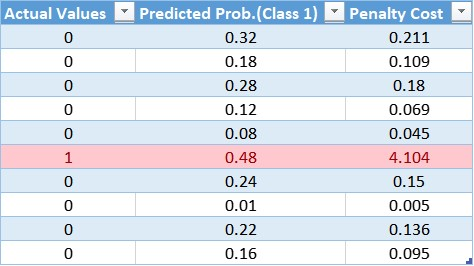Through the table, we can confirm the small weight applied to the cost function for the majority class that results in a smaller error value, and in turn, less update to the model coefficients. A more considerable weight value applied to the cost function for the minority class that results in a larger error calculation, and in turn, more updates to the model coefficients. This way, we can shift the bias of the model so that it could also reduce the errors of the minority class.

Conclusion:

• Small weights result in a small penalty and a small update to the model coefficients
• Large weights result in a large penalty and a large update to the model coefficients

## Implementation in Python

Here, we will be using the same heart stroke data for our predictions. First, we will train a simple logistic regression then we will implement the weighted logistic regression with class_weights as ‘balanced’. Finally, we will try to find the optimal value of class weights using a grid search. The metric we try to optimize will be the f1 score.

### 1. Simple Logistic Regression:

Here, we are using the sklearn library to train our model and we are using the default logistic regression. By default, the algorithm will give equal weights to both the classes.

The f1-score for the testing data: 0.0We got the f1 score as 0 for a simple logistic regression model. Looking at the confusion matrix, we can confirm that our model is predicting every observation as will not have a heart stroke. This model is not any better than the mode model that we have created earlier. Let’s try to add some weights to the minority class and see if that helps.

### 2. Logistic Regression (class_weight=’balanced’):

We have added the class_weight parameter to our logistic regression algorithm and the value we have passed is ‘balanced’.

The f1-score for the testing data: 0.10098851188885921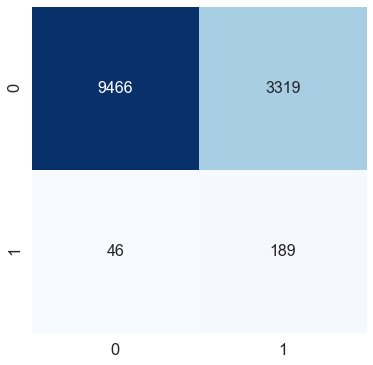By adding a single class weight parameter to the logistic regression function, we have improved the f1 score by 10 percent. We can see in the confusion matrix that even though the misclassification for class 0 (no heart stroke) has increased, the model can capture the class 1 (heart stroke) pretty well.

Can we improve the metric any further just by changing class weights?

### 3. Logistic Regression (manual class weights):

Finally, we are trying to find optimal weights with the highest score using grid search. We will search for weights between 0 to 1. The idea is, if we are giving n as the weight for the minority class, the majority class will get 1-n as the weights.

Here, the magnitude of the weights is not very large but the ratio of weights between majority and minority class will be very high.

Example:

w1 = 0.95

w0 = 1 – 0.95 = 0.05

w1:w0 = 19:1

So, the weights for the minority class will be 19 times higher than the majority class.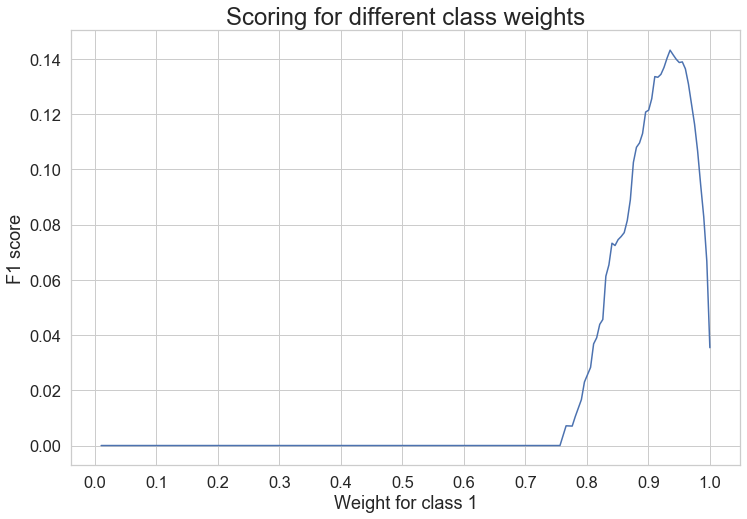Through the graph we can see that the highest value for the minority class is peaking at about 0.93 class weight.

Using grid search, we got the best class weight, i.e.  0.06467 for class 0 (majority class), 1: 0.93532 for class 1 (minority class).

Now that we have our best class weights using stratified cross-validation and grid search, we will see the performance on the test data.

The f1-score for the testing data: 0.1579371474617244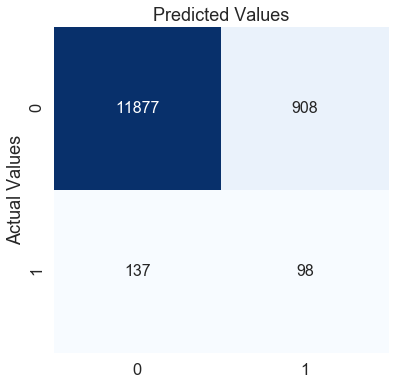By manually changing the values of the weights we are able to improve the f1-score further by 6% approximately. The confusion matrix also shows that from the previous model, we are able to predict the class 0 much better but at the cost of misclassification of our class 1. This is all dependent on the business problem or the error type you want to reduce more. Here our focus was to improve the f1 score and that we are able to do by just tweaking the class weights.

## Tips to improve scoring further

• Feature Engineering: For simplicity, we have used only the given independent variables. You can try creating new features like frequency-based, interaction-based, by grouping features, descriptive statistics based features, etc.
• Tuning the threshold: By default, the threshold is 0.5 for all algorithms. You can try different values for the threshold and can find the optimal value by using a grid search or randomize search
• Using Advanced Algorithms: For this explanation, we have used only logistic regression. You can try different advanced bagging and boosting algorithms. And finally can also try stacking or blending the multiple algorithms

## End Notes

I hope this article gave you a good idea about how class weights can help to handle a class imbalance problem and how easy it is to implement in python.

Although we have discussed how class weight works only for logistic regression, the idea remains the same for every other algorithm; it’s just the change of the cost function that each algorithm uses to minimize the error and optimize results for the minority class.

You can also read this article on our Mobile APP•Amandeep says:

Nice work ….

•Pranjal Sett says:

Regarding this, could you please explain a bit more:
For the values of the weights, we will be using the class_weights=’balanced’ formula.

w0= 10/(2*1) = 5

w1= 10/(2*9) = 0.55

w0 means “0” class and number of “0” class is 9 here, then why we are multiply with “1” here?

•Kamaldeep Singh says:

Hi Pranjal,
Thank you for noticing the mistake. I will go through it and will update it asap.

•Aslam Shah says:

very informative .. keep sharing